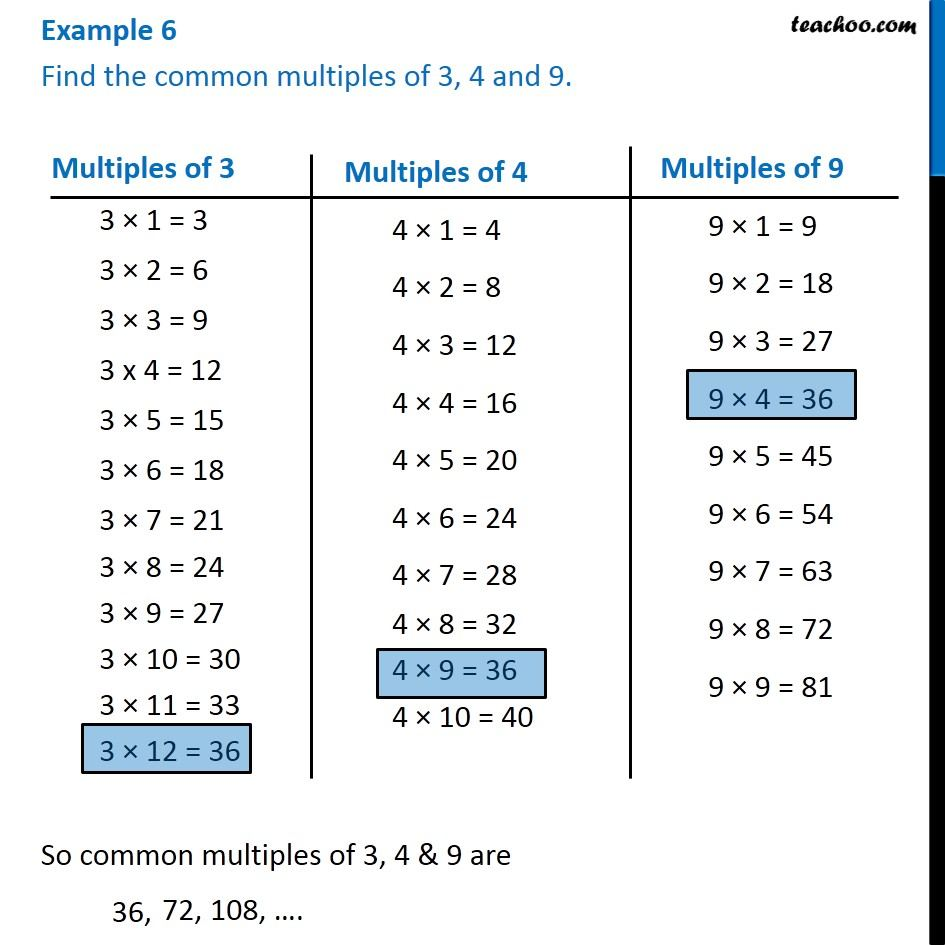Examples

Chapter 3 Class 6 Playing with Numbers
Serial order wiseLearn in your speed, with individual attention - Teachoo Maths 1-on-1 Class

### Transcript

Example 6 Find the common multiples of 3, 4 and 9.Multiples of 3 3 × 1 = 3 3 × 2 = 6 3 × 3 = 9 3 x 4 = 12 3 × 5 = 15 3 × 6 = 18 3 × 7 = 21 3 × 8 = 24 3 × 9 = 27 3 × 10 = 30 3 × 11 = 33 3 × 12 = 36 Multiples of 4 4 × 1 = 4 4 × 2 = 8 4 × 3 = 12 4 × 4 = 16 4 × 5 = 20 4 × 6 = 24 4 × 7 = 28 4 × 8 = 32 4 × 9 = 36 4 × 10 = 40 Multiples of 9 9 × 1 = 9 9 × 2 = 18 9 × 3 = 27 9 × 4 = 36 9 × 5 = 45 9 × 6 = 54 9 × 7 = 63 9 × 8 = 72 9 × 9 = 81 So common multiples of 3, 4 & 9 are 36,